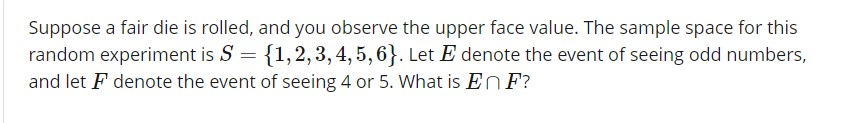# Suppose a fair die is rolled, and you observe the upper face value. The sample space for thisrandom experiment is S 11,2,3,4,5,63. Let E denote the event of seeing odd numbers,and let F denote the event of seeing 4 or 5. What is EnF?

Question
1 views

Hello. I appreciate any help. Thanks.help_outlineImage TranscriptioncloseSuppose a fair die is rolled, and you observe the upper face value. The sample space for this random experiment is S 11,2,3,4,5,63. Let E denote the event of seeing odd numbers, and let F denote the event of seeing 4 or 5. What is EnF? fullscreen
check_circle

star
star
star
star
star
1 Rating
Step 1

Intersection of events:

Consider two events A and B. The intersection of two events A and B is the collection of all outcomes that are the elements of both A and B and is denoted as AB.

Step 2

Here, the sample space for the random experiment is, S = {1, 2, 3, 4, 5, 6}.

The event E denotes the set of odd numbers and F denotes the event of seeing 4 or 5.

The set of all outcomes in the event E is, E = {1, 3, 5} and the set of all outcomes in the ev...

### Want to see the full answer?

See Solution

#### Want to see this answer and more?

Solutions are written by subject experts who are available 24/7. Questions are typically answered within 1 hour.*

See Solution
*Response times may vary by subject and question.
Tagged in

### Other